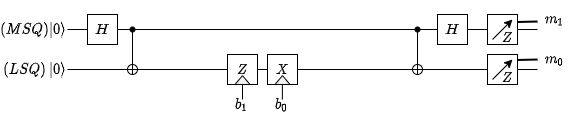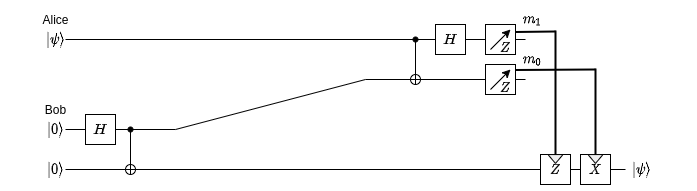# 5 Applications of Quantum Information

Quantum Computers have gained much popularity recently because of their potential to outperform their classical counterparts in a variety of tasks.

In this post I ‘ll briefly go over some of their applications in Computing and Communication that fascinated me.

## Quantum Algorithms

Quantum Computers process information that, contrary to classical computers, is encoded in qubits (quantum states) instead of bits (logical states). A qubit is a vector in the 2-dimensional complex vector space (Hilbert space). A quantum state of a system is described mathematically as the Kronecker product of the states of its qubits.

To understand the scaling effect induced by this difference in the vector spaces in which information is encoded, consider the following fact: to store the quantum state of a system with $$n$$ qubits, we need $$2^{n+1} - 2$$ classical bits. This means that in order to store the state of a 100-qubit quantum system, the whole classical memory currently available in the world is not enough.

Quantum algorithms consist of 5 steps:

1. Initialization of qubits
2. Apply Hadamard gates to create a superposition of the qubits
3. Apply some unitary transformation (this is where the algorithm is encoded)
4. Apply some processing of the result
5. Measure the qubits.

The second and third step is the quintessence of the quantum algorithm; it applies a transformation in all the possible states at once, and thus has potential to solve some problems superpolynomially or even exponentially faster than conventional computers.

The most famous quantum algorithms to date are Shor’s factoring algorithm, which offers a superpolynomial speedup in prime factorization and Grover’s search algorithm that speeds up unstructured database search by a polynomial factor.

## Quantum Random Number Generation (QRNG)

Generation of truly random values is extremely important for a plethora of applications (cryptography, statistics, lotteries, etc.). The probabilistic nature of quantum mechanics makes them ideal for this task.

When measuring qubits, the qubit collapses in one of the eigenvector states of the measurement basis, with a probability equal to the square of the norm of the corresponding eigenvalue.

Therefore, all we have to do to generate randomness is measure a qubit in a different basis, for example measure $$| 0 \rangle$$ in the $$X$$ basis; or superpose qubit $$| 0 \rangle$$ with a Hadamard gate $$H$$ and then measure on the $$Z$$ basis (which is more common). The qubit will collapse and the measuring equipment will give $$+1$$ or $$-1$$, each with a probability of $$0.5$$.

There are already companies that produce QRNG equipment.

## Quantum Key Distribution (QKD)

QKD is a way for two parties to exchange a cryptographic key. The protocol is the following (note that that $$|+\rangle$$ and $$|-\rangle$$ are eigenstates of the $$X$$ basis):

1. Bob picks randomly a basis $$B_i \in { X, Z }$$ and also picks randomly and privately a bit $$b_i \in { 0, 1 }$$
2. Bob prepares qubit $$\psi_i$$ according to:
$$B_i$$ $$b_i$$ $$\psi_i$$
$$Z$$ $$0$$ $$|0 \rangle$$
$$Z$$ $$1$$ $$|1 \rangle$$
$$X$$ $$0$$ $$|+ \rangle$$
$$X$$ $$1$$ $$|- \rangle$$
1. Bob sends qubit $$\psi_i$$ to Alice.
2. Alice measures qubit $$\psi_i$$ in a randomly picked basis $$\tilde{B}_i \in { X, Z }$$ and records the measurement outcome $$\tilde{m_i}$$.
3. Alice and Bob repeat steps 1 to 4 a total of $$N$$ times.
4. Alice and Bob publicly announce the N bases they each used.
5. Alice and Bob sift out the $$M \le N$$ runs in which the used the same basis and throw out the rest.
6. Alice and Bob randomly pick a subset of the sifted pairs $$(b_i, \tilde{m_i})$$ and compare them using a classical communication channel. If the outcomes correlate perfectly, they can confidently use the remaining ones as a shared private key.

After the key distribution, Alice and Bob can use it with whichever cryptographic algorithm they want.

To prevent an eavesdropper to get partial information on the key, there are various (classical) methods such as Information Reconciliation and Privacy Amplification.

There are already commercial security products that implement QKD.

## Superdense coding

Superdense coding allows us to reliably transmit two bits of information using only one qubit.

Say we have Bob and Alice again. They start with a pair of entangled qubits (specifically a Bell pair). The LSQ (least-significant-qubit) is sent to Bob, who applies $$Z$$ to it, if his MSB is 1 and $$X$$ if his LSB is 1.

The the MSQ is sent back to Alice, who performs a Bell measurement.Alice can find out what Bob’s MSB ($$b_1$$) and LSB ($$b_0$$) were via the following table:

$$b_1$$ $$b_0$$ $$m_1$$ $$m_0$$
$$0$$ $$0$$ $$+1$$ $$+1$$
$$0$$ $$1$$ $$+1$$ $$-1$$
$$1$$ $$0$$ $$-1$$ $$+1$$
$$1$$ $$1$$ $$-1$$ $$-1$$

## Quantum Teleportation

Quantum Teleportation is a setup that allows us to transfer the state of one qubit to another one, possibly far away, without direct interaction between them.

Let’s say Alice wants to send to Bob her qubit’s state. Bob starts with a Bell pair, he sends to Alice his MSQ, Alice measures it with the state she wants to send and communicates (classically) the measurement results back to Bob. Bob then applies $$Z$$ or $$X$$ gates to his LSQ according to these results, and after that his MSQ will have the same state as the (now collapsed) qubit of Alice.Well it sounds cooler than it is, but it is actually very useful.

Since quantum states cannot be cloned, quantum teleportation is an effective way to at least move them around. A potential application of quantum teleportation would be in quantum repeater nodes. Think of routing, but for qubits. This will enable the engineering of the Quantum Internet.